# Python 一网打尽＜排序算法＞之先从玩转冒泡排序开始## 2. 冒泡排序算法

• 摆擂台法
• 相邻的两个数字比较法

### 2.1 摆擂台法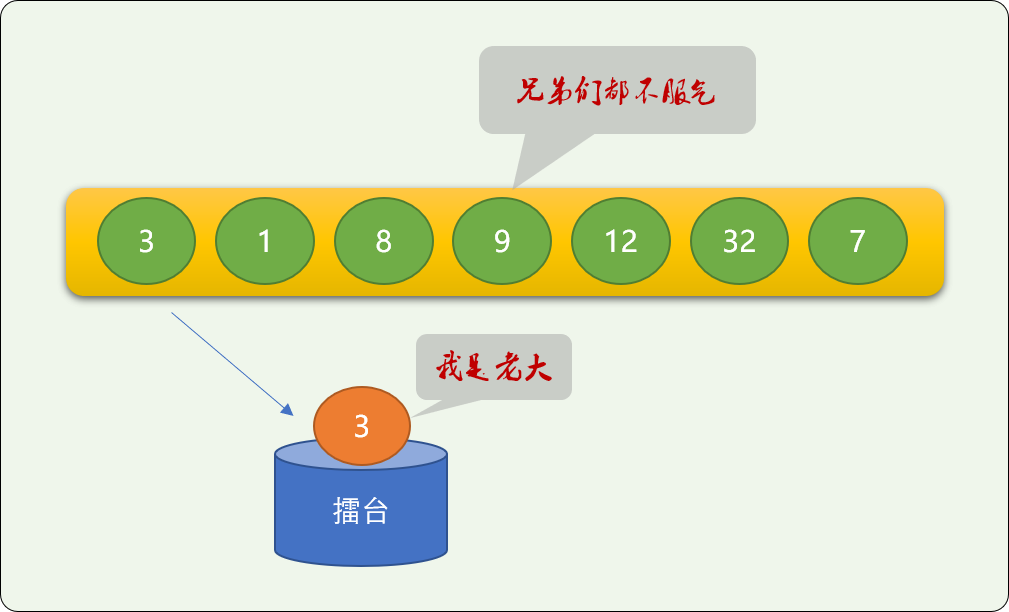``````nums = [3, 1, 8, 9, 12, 32, 7]
# 第一个数字登上擂台
m = nums
# 其它数字不服气
for i in range(1, len(nums)):
# PK 过程中，大的留在擂台上
if nums[i] > m:
m = nums[i]
# 最后留在擂台上的就是最大值
print("最大值是:", m)
``````

``````nums = [3, 1, 8, 9, 12, 32, 7]
# 第一个数字登上擂台
ms=[]
for _ in range(len(nums)):
m = nums
for i in range(1, len(nums)):
if nums[i] > m:
m = nums[i]
# 依次找到的最大值存入新数列
ms.append(m)
# 从原数列中移出找到的最大值，下一轮要在没有它的数列中重新找，不移走，无论找多少次，还会是它
nums.remove(m)
print(ms)
'''

[32, 12, 9, 8, 7, 3, 1]
'''
``````

• 另开辟了新空间，显然空间复杂度增加了。
• 原数列的最大值找到后就删除了，目的是不干扰余下数字继续查找最大值。当对所有数字排好序后，原数列也破坏了

``````nums = [3, 1, 8, 9, 12, 32, 7]
# 第一个数字登上擂台
nums_len = len(nums)
for _ in range(len(nums)):
m = nums
for i in range(1, nums_len):
if nums[i] > m:
m = nums[i]
# 最大值找到，移动最后
nums.remove(m)
nums.append(m)
# 这个很关键，缩小原数列的结束位置
nums_len = nums_len - 1
print(nums)
'''

[32, 12, 9, 8, 7, 3, 1]
'''
``````

### 2.2 相邻两个数字相比较

``````nums = [3, 1, 8, 9, 12, 32, 7]
for i in range(len(nums)-1):
# 相邻 2 个数字比较
if nums[i] > nums[i + 1]:
# 如果前面的数字大于后面的数字，则交换
nums[i], nums[i + 1] = nums[i + 1], nums[i]
# 显然，数列最后位置的数字是最大的
print(nums[len(nums) - 1])
'''

32
'''
``````

``````nums = [3, 1, 8, 9, 12, 32, 7]
# 外层重复的 100 意味着找了 100 次最大值，这里只是说明问题，就是不停找最大值，显然，是用不着找100 次的
for j in range(100):
for i in range(len(nums)-1):
# 相邻 2 个数字比较
if nums[i] > nums[i + 1]:
# 如果前面的数字大于后面的数字，则交换
nums[i], nums[i + 1] = nums[i + 1], nums[i]

print(nums)
``````

``````nums = [3, 1, 8, 9, 12, 32, 7]
# 找多少次最大值，数列长度减 1
for j in range(len(nums)-1):

for i in range(len(nums)-1-j):
# 相邻 2 个数字比较
if nums[i] > nums[i + 1]:
# 如果前面的数字大于后面的数字，则交换
nums[i], nums[i + 1] = nums[i + 1], nums[i]

print(nums)
``````

## 3. 选择排序算法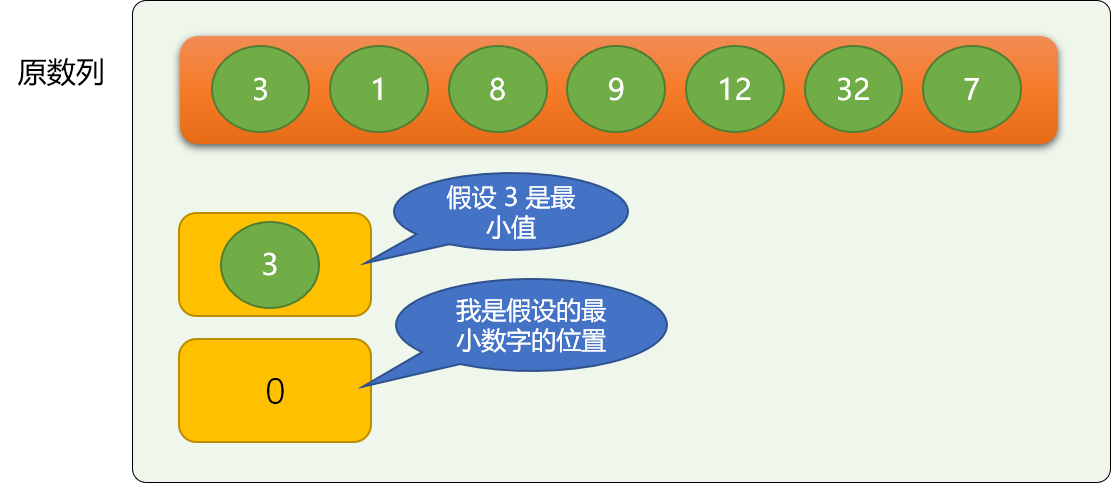``````nums = [6, 2, 5, 9, 12, 1, 7]
# 擂台！假充第一 个数字是最小值
mi = nums
# 假设的最小数字位置
mi_idx = 0
# 真正最小数字的位置
real_idx = mi_idx
for i in range(mi_idx + 1, len(nums)):
if nums[i] < mi:
mi = nums[i]
# 记住更小数字的位置，不记着交换
real_idx = i
# 如有更小的
if real_idx != mi_idx:
# 交换
nums[real_idx], nums[mi_idx] = nums[mi_idx], nums[real_idx]
print(nums)
'''

[1, 2, 5, 9, 12, 6, 7]
'''
``````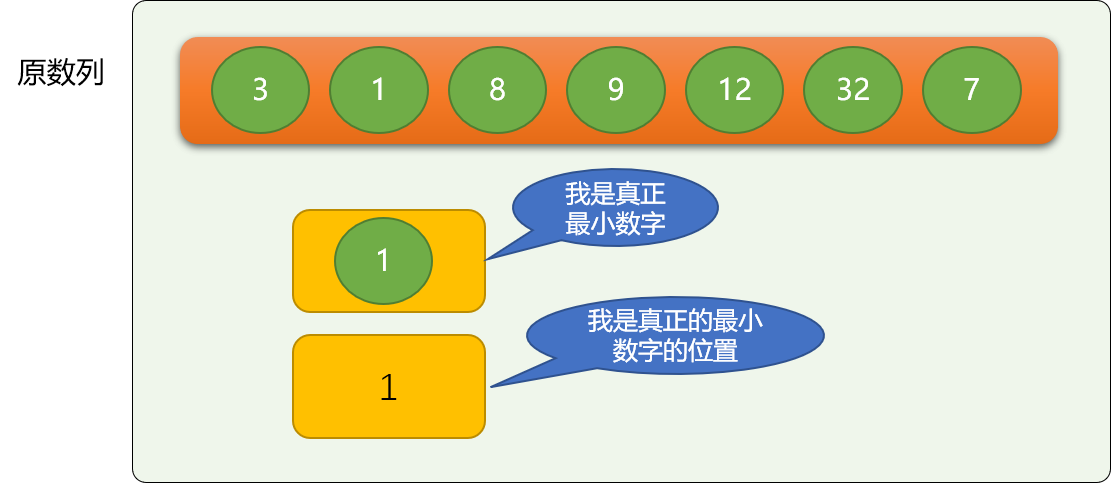``````nums = [6, 2, 5, 9, 12, 1, 7]
for j in range(len(nums)-1):
mi = nums[j]
# 假设的最小数字位置
mi_idx = j
# 真正最小数字的位置
real_idx = mi_idx
for i in range(mi_idx + 1, len(nums)):
if nums[i] < mi:
mi = nums[i]
# 记住更小数字的位置
real_idx = i
# 如有更小的
if real_idx != mi_idx:
# 交换
nums[real_idx], nums[mi_idx] = nums[mi_idx], nums[real_idx]
print(nums)
'''

[1, 2, 5, 6, 7, 9, 12]
'''
``````

## 4. 插入排序

• 把原数列从逻辑(根据起始位置和结束位置在原数列上划分)上分成前、后两个数列，前面的数列是有序的，后面的数列是无序的。

刚开始时，前面的数列（后面简称前数列）只有唯一的一个数字，即原数列的第一个数字。显然是排序的！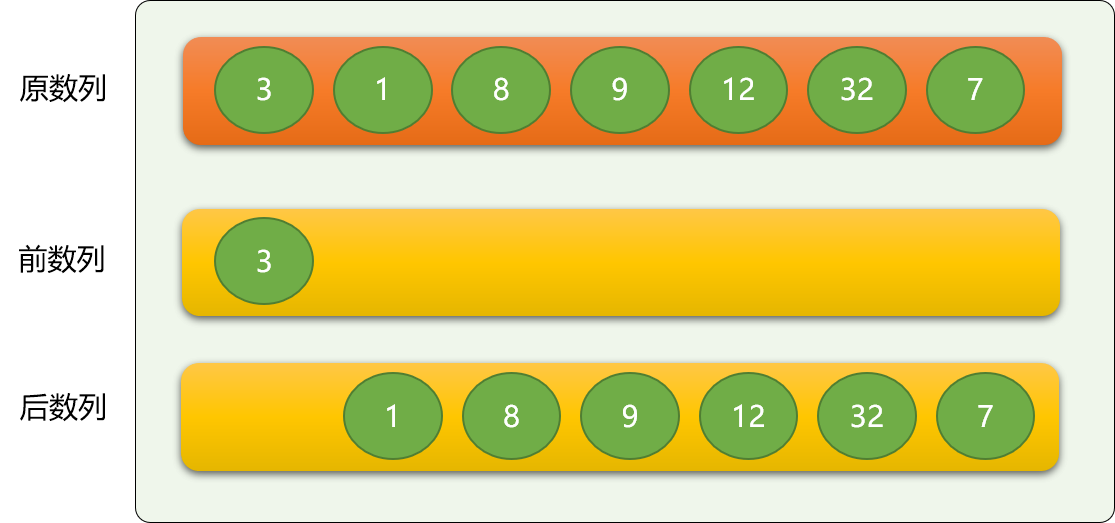• 依次从后数列中逐个拿出数字，与前数列的数字进行比较，保证插入到前数列后，整个前数列还是有序的。

如上，从后数列中拿到数字 1 ，然后与前数字的 3 进行比较，如果是从大到小排序，则 1 就直接排到 3 后面，如果是从小到大排序，则 1 排到 3 前面。

这里，按从小到大排序。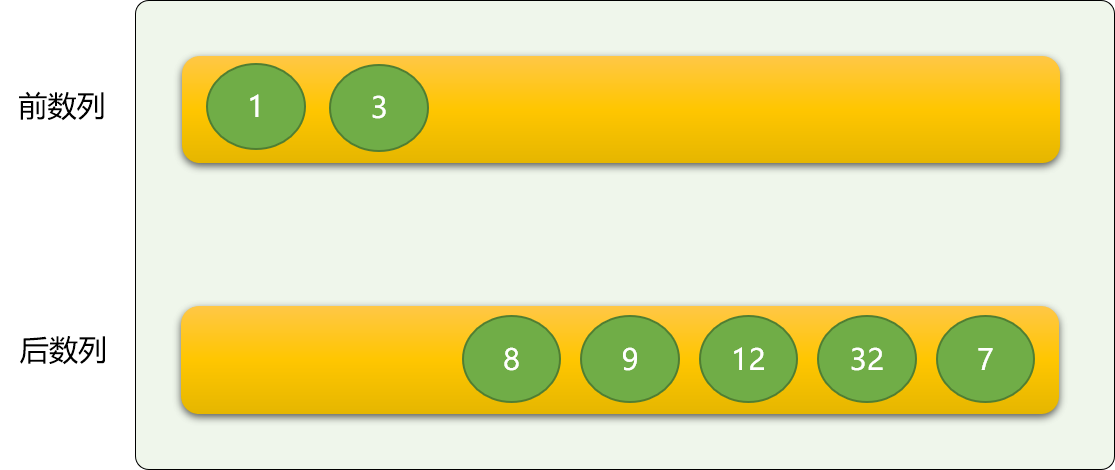• 比较： 后数列的数字要与前数字的数字进行大小比较，这个与冒泡和选择排序没什么不一样。
• 移位： 如果前数列的数字大于后数列的数字，则需要向后移位。也可以和冒泡排序一样交换。
• 插入： 为后数列的数字在前数列中找到适当位置后，插入此数据。

• 前指针用来在前数列中定位数字，方向是从右向左。

• 后指针用来在后数字中定位数字，方向是从左向右。

前指针初始的位置之前为前数列，后指针初始时的位置为后数列。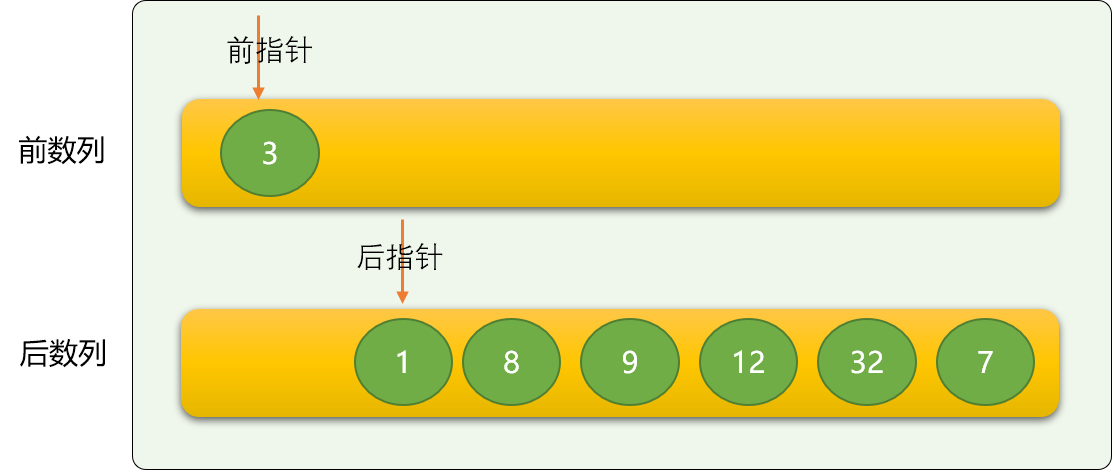``````nums = [3, 1, 8, 9, 12, 32, 7]

# 后指针指向原数列的第 2 个数字,所以索引号从 1 开始
for back_idx in range(1, len(nums)):
# 前指针和后指针的关系，
front_idx = back_idx - 1

# 临时变量，比较时，前数列的数字有可能要向后移位，需要把后指针指向的数字提前保存
tmp = nums[back_idx]

# 与前数列中的数字比较
while front_idx >= 0 and tmp < nums[front_idx]:
# 移位
nums[front_idx + 1] = nums[front_idx]
front_idx -= 1
if front_idx != back_idx - 1:
# 插入
nums[front_idx + 1] = tmp

print(nums)
'''

[1,3,7,8,9,12,32]
'''
``````

``````nums = [3, 1, 8, 9, 12, 32, 7]
for back_idx in range(1, len(nums)):
for front_idx in range(back_idx, 0, -1):
if nums[front_idx] < nums[front_idx - 1]:
nums[front_idx], nums[front_idx - 1] = nums[front_idx - 1], nums[front_idx]
else:
break
print(nums)
``````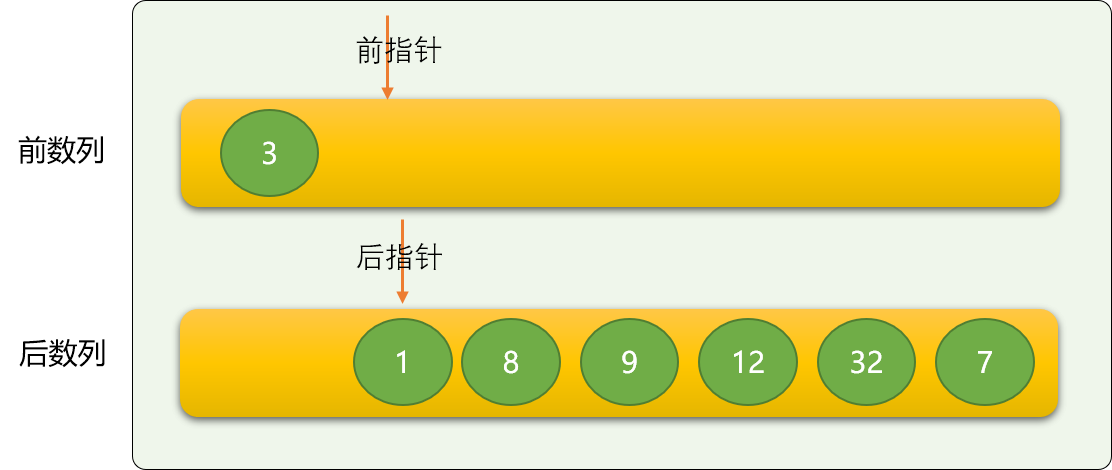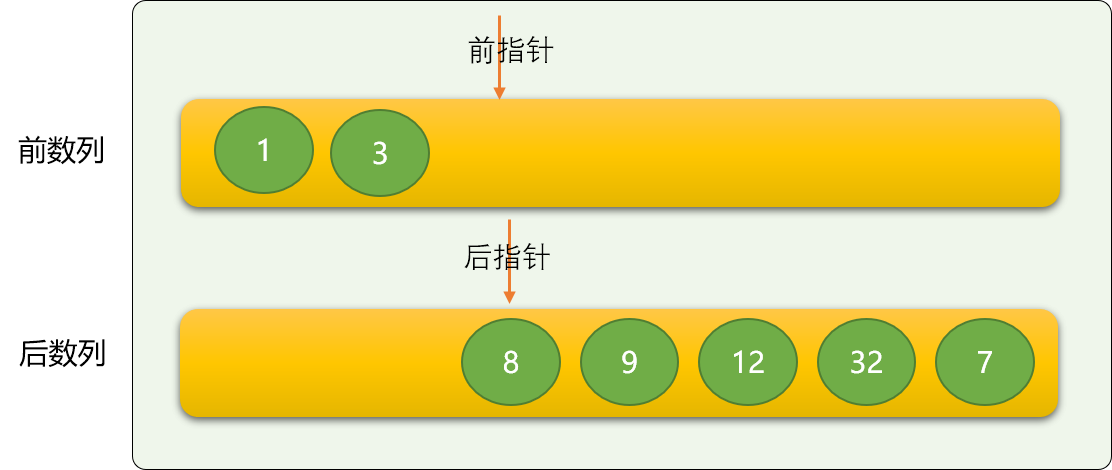## 5. 快速排序

• 分治思想： 全局到局部、或说是粗糙完美的逐步细化过程。

类似于画人物画。

先绘制一个轮廓图，让其看起来像什么！

然后逐步细化，让它真的就是什么！

快速排序也是这个思想，刚开始，让数列粗看起来有序，通过逐步迭代，让其真正有序。

• 二分思想： 在数列选择一个数字（基数）为参考中心，数列比基数大的，放在左边（右边），比基数小的，放在右边（左边）。

第一次的二分后：整个数列在基数之上有了有序的轮廓，然后在对基数前部分和后部分的数字继续完成二分操作。

• 左指针初始指向最左边数字。
• 右指针初始指向最右边数字。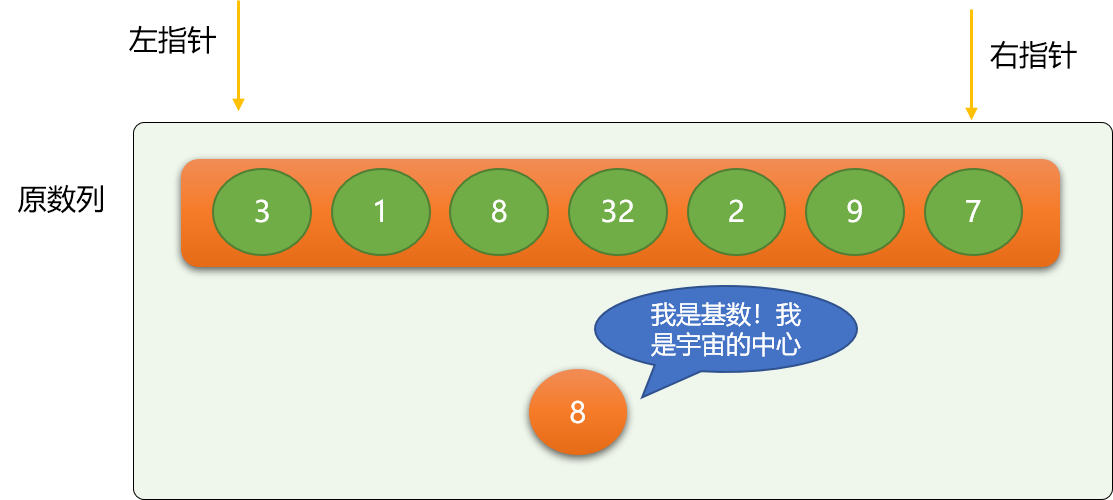• 左指针不停向右移动，至到遇到大于等于基数的数字 ，同理右指针不停向左移动，至到碰到小于等于基数的数字。
• 交换左指针和右指针的位置的数据。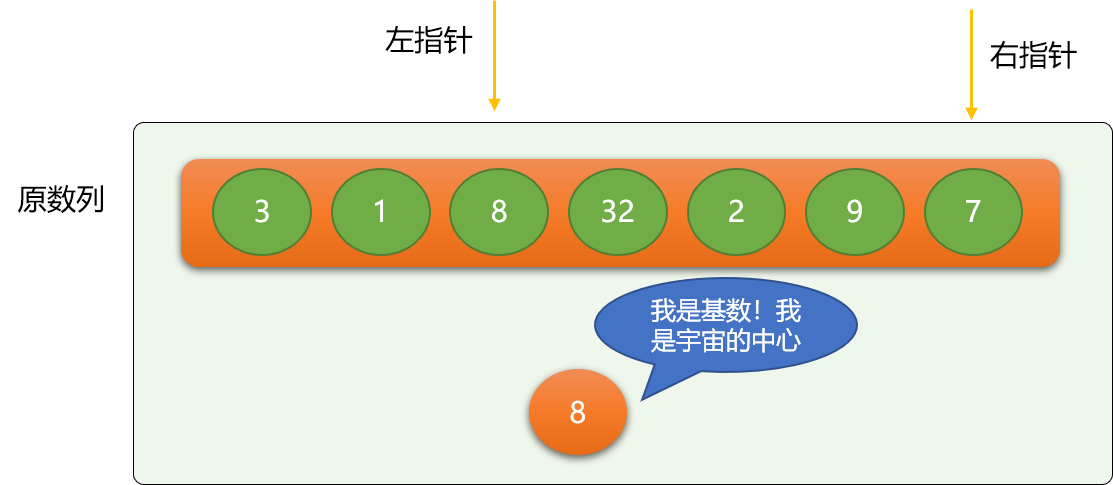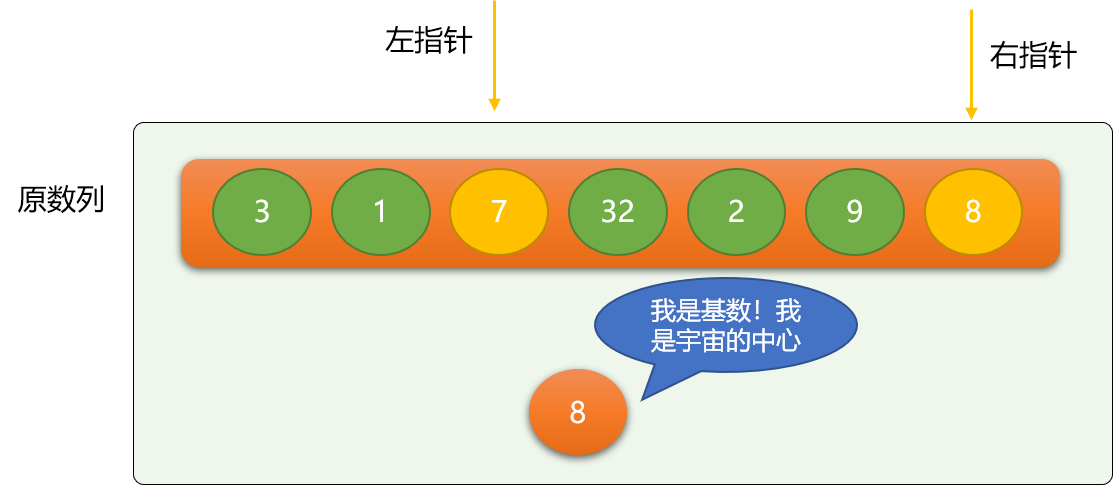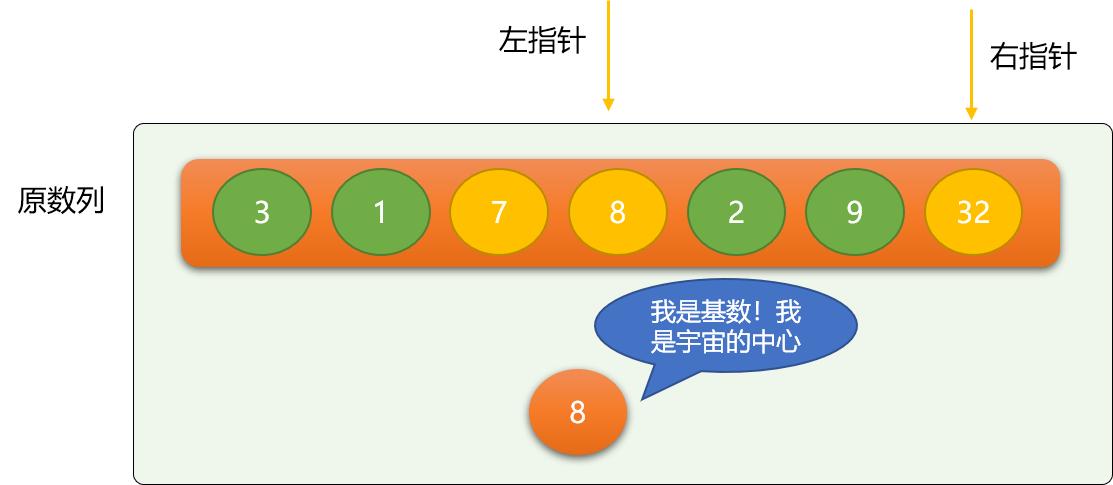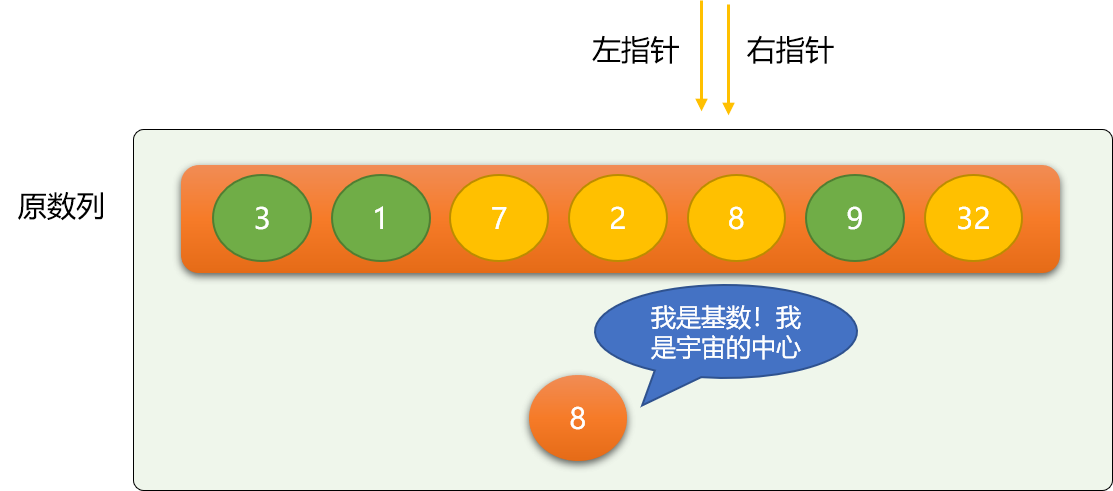``````nums = [3, 1, 8, 32, 2, 9, 7]

def quick_sort(nums):
# 左指针
left = 0
# 右指针
right = len(nums) - 1
# 基数，可以是任意数字，一般选择数列的第一个数字
base_n = 8
while left < right:
# 左指针向右移动，至到时左指针位置数字大于等于基数，
while nums[left] < base_n and left < right:
left += 1
while nums[right] > base_n and right > left:
right -= 1
# 交换
nums[left], nums[right] = nums[right], nums[left]

quick_sort(nums)
print(nums)
``````

``````[3, 1, 7, 2, 8, 9, 32]
``````

``````nums = [3, 1, 8, 32, 2, 9, 7]

def quick_sort(nums, start, end):
if start >= end:
return
# 左指针
left = start
# 右指针
right = end
# 基数
base_n = nums[start]
while left < right:
while nums[right] > base_n and right > left:
right -= 1
# 左指针向右移动，至到时左指针位置数字大于等于基数，
while nums[left] < base_n and left < right:
left += 1
# 交换
nums[left], nums[right] = nums[right], nums[left]
# 左边数列
quick_sort(nums, start, left - 1)
# 右边数列
quick_sort(nums, right + 1, end)

quick_sort(nums, 0, len(nums) - 1)
print(nums)
'''

[1, 2, 3, 7, 8, 9, 32]
'''
``````

## 6. 总结

4 total views,  1 views today